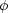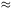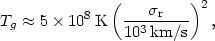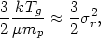5.3.2. Infall and compressional heating

The heating of the intracluster gas will now be considered. The major point of this discussion is that, although the gas is quite hot, no major ongoing heating of the gas is generally necessary. This is true because the cooling time in the gas is long (equation 5.23), and the thermal energy in the gas is comparable to or less than its gravitational potential energy. Almost any method of introducing the gas into the cluster, either from outside the cluster or from galaxies within the cluster, will heat it to temperatures on the order of those observed.

Heating of the gas due to infall into the cluster and compression will be considered here. First, imagine that the cluster was formed before the intracluster gas fell into the cluster, and that the intracluster gas makes a negligible contribution to the mass of the cluster (Section 4.4). If the gas was initially cold, and located at a large distance from the cluster, then its initial energy can be ignored. If the cluster potential remains fixed while the gas falls into the cluster and the gas neither loses energy by radiation nor exchanges its energy with other components of the cluster, then the total energy of the gas will remain zero. After falling into the cluster, the gas will collide with other elements of gas, and its kinetic energy will be converted to thermal energy. Thus infall and compression can produce temperatures on the order of(5.24)

whereis the gravitational potential in the cluster. At the center of an isothermal cluster,-9r2, wherer is the line-of-sight velocity dispersion of the cluster. If this is substituted in equation (5.24), the derived temperature is(5.25)

which is a factor of 5-10 times larger than the observed temperatures (equation 4.10). Of course, the gas that falls into a cluster was presumably bound to the cluster before it fell in, so that equation (5.25) overestimates the temperature. A similar calculation for gas bound to the cluster is given in Shibazaki et al. (1976).

The temperature may also be lower because of cooling during the infall, or because the gas fell in at the same time that the cluster was forming and thus experienced a smaller potential on average. If the gas fell in at the same time that the cluster collapsed and was heated by the rapid variation of the potential during violent relaxation (Section 2.9.2), then it might have the same energy per unit mass as the matter in galaxies,(5.26)

which gives equation (5.14) for the temperature. This is in reasonable agreement with the intracluster gas temperatures determined from X-ray spectra (equation 4.10).

These crude estimates are meant only to illustrate the point that the observed gas temperatures are consistent with heating due to infall into the cluster. More detailed models for infall are discussed in Section 5.10.1.